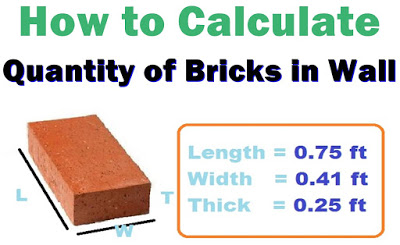# How Many Bricks per 1 M2 / How Many Bricks Per Square Metre

## How Many Bricks per 1 M2 / How Many Bricks Per Square Metre;

Here is How To Calculate

### Number of Bricks in 1 Square Metre (M2)How to calculate the number of bricks in 1  square foot.

#### Size of brick:(adsbygoogle = window.adsbygoogle || []).push({});

Length of Bricks in feets (L) = 9” = 0.75 ft

Width of Bricks in feets (W) = 3” = 0.41 ft

Thickness of Bricks in feets (T) =2” = 0.25 ft

Area of  a brick = Length of brick x Width of brick

Area of  each Brick in square foot = L x W

= 0.75 x 0.41

= 0.3075 ft2

No. of bricks per 1 SQF = 1 square foot of brickwork/ Area of 1 brick

Numbers of bricks in 1 square foot = 1/(0.3075) =3.25 bricks

Mortar covered 10 % space so

=3.25 x (10/100)

=0.325

Number/Quantity of bricks in 1 Square Foot = 3.25 – 0.325=2.925 bricks

#### Number of Bricks in 1 Square Metre M2

1 Square Metre =  10.7 square foot

1square foot = 2.925 Bricks

10.7 Square foot = ?

=2.925*10.7/1

= 31.3 Bricks

We  then get  31.3 numbers of bricks required in 1 M2.

### Check>> How to Calculate The Number of Mabati for a House

#### Related Posts

Select currency
USD United States (US) dollar
error: Content is protected !!
0